1. Worksheets>
2. Math>
3. Pre-Algebra>
4. Integers>
5. Compare and Order

Compare and Order the Integers Worksheets

Comparing and ordering integers' worksheets have a variety of activities to compare the integers. Real-life word problems are included. Ample exercises to order integers in the increasing and decreasing order are also here for practice.

Cut and glue activity: Famished animals

Cut out the famished animals (graphics) from the bottom of the worksheet and glue them in the appropriate boxes to compare the integers.Comparing integers using symbols

Compare each pair of integers using the greater than, lesser than or equal to symbol. Six exclusive worksheets are here for practice.Level 1:

Level 2:

Comparing and ordering integers

Compare and write single digit and two-digit positive and negative integers in the increasing or decreasing order based on the symbols.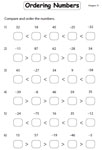Ordering integers: Standard

Compare the integers and arrange them in the increasing or decreasing order.Ordering integers with word problem

In section A, order the set of integers in the increasing order and in section B order them in the decreasing order. Interesting word problem included.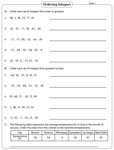Greater vs Smaller

Colorful fish are kept inside a fishbowl. Circle the fish which carries the greatest integer in section 1 and the smallest integer in section 2.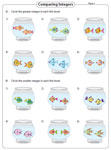Greatest vs Smallest

A set of integers are given. Circle the greatest integer among them in section A and smallest integer in section B. Word problems are given in section C and section D.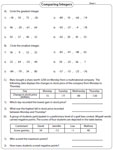Comparing temperatures: Thermometer

Two thermometers recording different temperatures are shown. Compare each pair of thermometers and choose the correct option from the choices given.Comparing integers: Addition and subtraction

Involve the addition or subtraction operation and compare the integers on either side. Each worksheet carries 16 problems.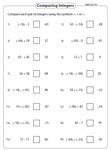Easy:

Moderate:

Comparing integers: Multiplication & Division

Perform the multiplication or division operation in each problem and compare the integers. Plentiful problems are given for practice.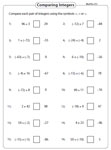Easy:

Moderate:

Comparing integers: Word problems

Integer word problems involving real-life scenarios are given in these worksheets. Read the data and answer the questions.The whole batch of worksheets on comparing and ordering integers can be downloaded instantly.

Related Worksheets